# How much heat is required to vaporize 100.0 g of ethanol, C2H,OH, at its boiling point?...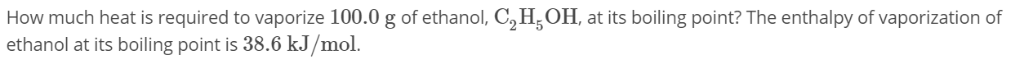How much heat is required to vaporize 100.0 g of ethanol, C2H,OH, at its boiling point? The enthalpy of vaporization of ethanol at its boiling point is 38.6 kJ/mol.

This Homework Help Question: "How much heat is required to vaporize 100.0 g of ethanol, C2H,OH, at its boiling point?..." No answers yet.

We need 10 more requests to produce the answer to this homework help question. Share with your friends to get the answer faster!

0 /10 have requested the answer to this homework help question.

Once 10 people have made a request, the answer to this question will be available in 1-2 days.
All students who have requested the answer will be notified once they are available.

#### Earn Coin

Coins can be redeemed for fabulous gifts.

Similar Homework Help Questions
• ### 222.The enthalpy of vaporization for chlorine is 20.41kJ/mol. How much heat is required to vaporize 293.39g...

222.The enthalpy of vaporization for chlorine is 20.41kJ/mol. How much heat is required to vaporize 293.39g of chlorine liquid at its boiling point? Report your answer in units of kJ.

• ### How much heat (in kJ) is required to raise the temperature of 202.8 g of ethanol...

How much heat (in kJ) is required to raise the temperature of 202.8 g of ethanol (mw=46.07) from 40.34 °C to its boiling point of  78.37 °C and then vaporize it completely creating ethanol gas at the boiling temperature? (specific heat = 2.46 J/g°C, ΔHvap = 48.6 kJ/mol)

• ### How much heat (in kJ) is required to raise the temperature of 288.7 g of ethanol...

How much heat (in kJ) is required to raise the temperature of 288.7 g of ethanol (mw=46.07) from 39.38 °C to its boiling point of 78.37 °C and then vaporize it completely creating ethanol gas at the boiling temperature? (specific heat = 2.46 J/g°C, ΔHvap = 48.6 kJ/mol)

• ### How much heat (in kJ) is required to raise the temperature of 124.9 g of ethanol...

How much heat (in kJ) is required to raise the temperature of 124.9 g of ethanol (mw=46.07) from 30.24 °C to its boiling point of  78.37 °C and then vaporize it completely creating ethanol gas at the boiling temperature? (specific heat = 2.46 J/g°C, ΔHvap = 48.6 kJ/mol)

• ### How much heat energy is required to convert 15.0 g of solid ethanol at -114.5 °C...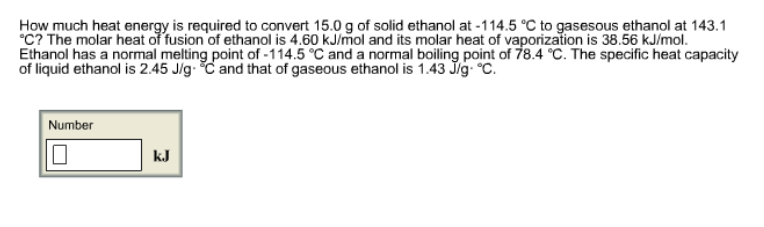How much heat energy is required to convert 15.0 g of solid ethanol at -114.5 °C to gasesous ethanol at 143.1 °C? The molar heat of fusion of ethanol is 4.60 kJ/mol and its molar heat of vaporization is 38.56 kJ/mol. Ethanol has a normal melting point of -114.5 °C and a normal boiling point of 78.4 °C. The specific heat capacity of liquid ethanol is 2.45 J/g·°C and that of gaseous ethanol is 1.43 J/g·°C. How much heat energy...

• ### QUESTION 3 How much heat (in kJ) is required to raise the temperature of 122 g of ethanol (mw 46.07) from 11.29 °C to i...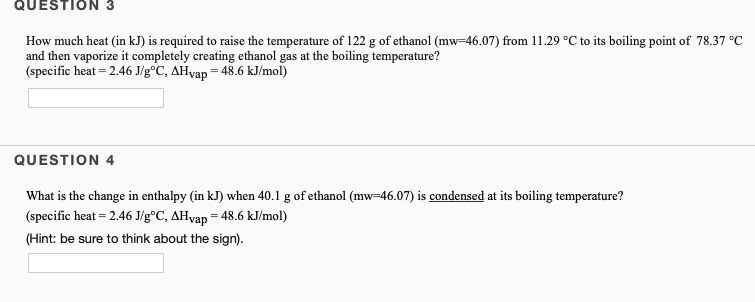QUESTION 3 How much heat (in kJ) is required to raise the temperature of 122 g of ethanol (mw 46.07) from 11.29 °C to its boiling point of 78.37 °C and then vaporize it completely creating ethanol gas at the boiling temperature? (specific heat - 2.46 J/g°C, AHyap = 48.6 kJ/mol) QUESTION 4 What is the change in enthalpy (in kJ) when 40.1 g of ethanol (mw-46.07) is condensed at its boiling temperature? (specific heat 2.46 J/g°C, AHvap 48.6 kJ/mol)...

• ### How much energy is required to vaporize 158 g of butane (C4H10) at its boiling point,...

How much energy is required to vaporize 158 g of butane (C4H10) at its boiling point, if its ΔHvap is 24.3 kJ/mol

• ### a. How much heat (kJ), at 1 atm, is required to raise the temperature of 25.0...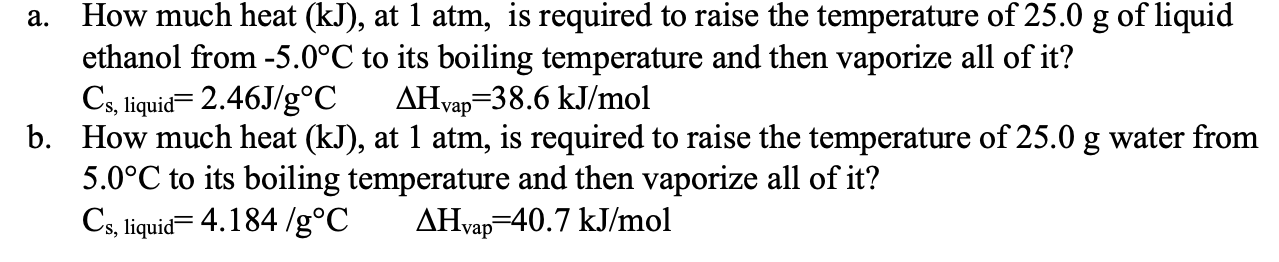a. How much heat (kJ), at 1 atm, is required to raise the temperature of 25.0 g of liquid ethanol from -5.0°C to its boiling temperature and then vaporize all of it? Cs, liquid= 2.46J/g°C AHvap=38.6 kJ/mol b. How much heat (kJ), at 1 atm, is required to raise the temperature of 25.0 g water from 5.0°C to its boiling temperature and then vaporize all of it? Cs, liquid=4.184 /g°C AHvap=40.7 kJ/mol

• ### How much heat energy is required to convert 19.8 g of solid ethanol at –114.5 °C...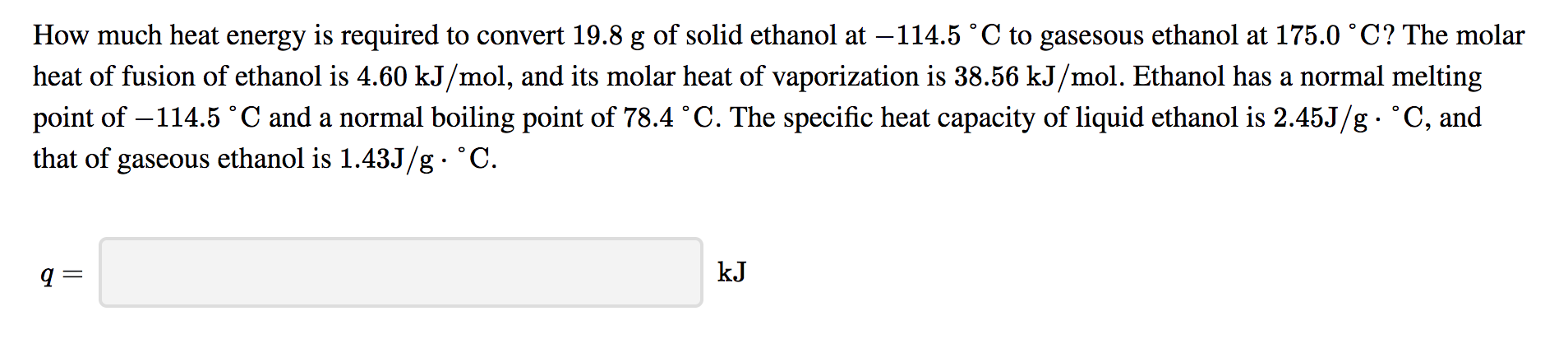How much heat energy is required to convert 19.8 g of solid ethanol at –114.5 °C to gasesous ethanol at 175.0 °C? The molar heat of fusion of ethanol is 4.60 kJ/mol, and its molar heat of vaporization is 38.56 kJ/mol. Ethanol has a normal melting point of –114.5 °C and a normal boiling point of 78.4 °C. The specific heat capacity of liquid ethanol is 2.45J/g • °C, and that of gaseous ethanol is 1.43J/g. °C.

• ### How much heat energy is required to convert 49.7 g of solid ethanol at –114.5 °C...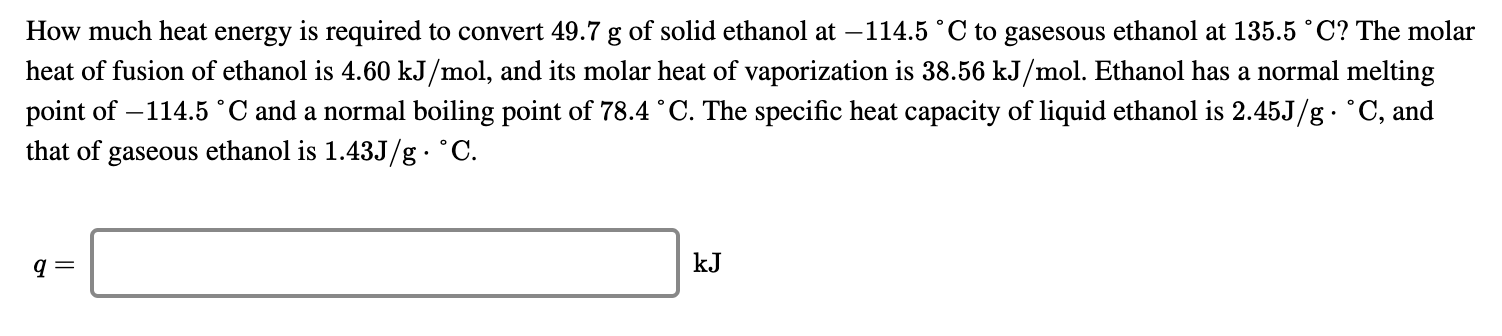How much heat energy is required to convert 49.7 g of solid ethanol at –114.5 °C to gasesous ethanol at 135.5 °C? The molar heat of fusion of ethanol is 4.60 kJ/mol, and its molar heat of vaporization is 38.56 kJ/mol. Ethanol has a normal melting point of -114.5 °C and a normal boiling point of 78.4 °C. The specific heat capacity of liquid ethanol is 2.45J/g. °C, and that of gaseous ethanol is 1.43J/g • °C. kᎫ